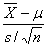# Statistics Hypothesis

## Sample: Hypothesis Tests

Question:

At recent times, the airlines have received complaints from passengers regarding their e-tickets, particularly when connecting flights and a change of airlines were involved. To investigate the problem an independent watchdog agency contacted a random sample of 20 airports and collected information on the number of complaints the airport had with e-tickets for the month of March. The information is reported below:

14 12 14 15 16 15 12 14 12 13 14 13 13 12 16 13 15 10 14 13

At the .05 significance level can the watchdog agency conclude the mean number of complaints per airport is less than 15 per month?

a. What assumption is necessary before conducting a test of hypothesis?
b. Plot the number of complaints per airport in a frequency distribution or a dot plot. Is it reasonable to conclude that the population follows a normal distribution?
c. Conduct a test of hypothesis and interpret the results.
****Calculate it in minitab too.

Solution:
a. What assumption is necessary before conducting a test of hypothesis?

The assumptions for the one-sample t-test are
Measurements are interval scale or better
Observations are independent of each other
The scores in the parent population are normally distributed.

b. Plot the number of complaints per airport in a frequency distribution or a dot plot. Is it reasonable to conclude that the population follows a normal distribution?It is reasonable to conclude that the population follows a normal distribution.

c. Conduct a test of hypothesis and interpret the results.

Null Hypothesis:

H0: m = 15
Alternative Hypothesis:
H1: m < 15

Since the sample size is (n = 20 < 30) small, we can test the above hypothesis by the use of small sample test (t-test) as follows:

Test Statistic:

t =We obtain the result using Minitab
One-Sample T: C1

Test of mu = 15 vs < 15

95% Upper
Variable N Mean StDev SE Mean Bound T P
C1 20 13.500 1.504 0.336 14.082 -4.46 0.000

Conclusion:
At α=0.05, since the p value is < 0.05, we reject the null hypothesis, thus we conclude that the mean number of complaints per airport is less than 15 per month.